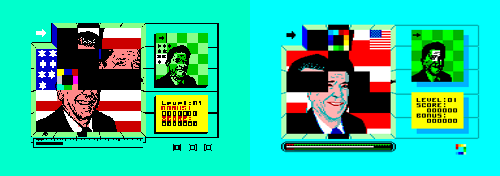# Shuffle an image with BitmapData – Part 2

December 7th update: part 3 released

This prototype somehow continues the Shuffle an image with BitmapData tutorial.

But the real aim of this code is beginning to create a game like Split Personalities, a game released for the Spectrum in 1986The game play revolves around assembling a number of pictures of famous people from the eighties which include Ronald Reagan, Margaret Thatcher, Charles & Diana and Clive Sinclair amongst others. Each famous face is split into a number of square pieces which are fed into the frame one at a time, on demand but in a random order. All you have to do is assemble the face by correctly positioning all the pieces in the frame! Complete a face to move on to the next level.

In my version, I am going to replace the famous people pictures with famous paintings.

So, move the square over the “fire” tile to release a piece of the painting to the right, then drag/drop it around the game field.

At the moment, the gameplay ends here but… hey… it’s just a 127 lines prototype… let me code another… let’s say 255 lines and you’ll enjoy playing it.

Really

Meanwhile, this is the actionscript that moves everything (sorry, it’s still uncommented and unexplained but I’ll release a complete tutorial as usual, meanwhile try to understand how does it work)

```import flash.display.BitmapData;
import flash.geom.Rectangle;
import flash.geom.Point;
Array.prototype.shuffle = function() {
for (x=0; x<24; x++) {
var from = Math.floor(Math.random()*25);
var to = this[x];
this[x] = this[from];
this[from] = to;
}
};
_root.createEmptyMovieClip("big_pic_obj",_root.getNextHighestDepth());
big_pic_obj.attachBitmap(big_picture,_root.getNextHighestDepth());
big_pic_obj._visible = false;
sequence = new Array(0, 1, 2, 3, 4, 5, 6, 7, 8, 9, 10, 11, 12, 13, 14, 15, 16, 17, 18, 19, 20, 21, 22, 23, 24);
sequence.shuffle();
field = new Array();
picture_in = 0;
_root.createEmptyMovieClip("tiles",_root.getNextHighestDepth());
_root.attachMovie("border","border",_root.getNextHighestDepth());
_root.attachMovie("fire","fire",_root.getNextHighestDepth());
border.onEnterFrame = function() {
this.gotoAndStop(1);
if (!dragging) {
this._x = Math.floor(_root._xmouse/100)*100;
this._y = Math.floor(_root._ymouse/100)*100;
}
else {
dist_x = _root._xmouse-save_x;
dist_y = _root._ymouse-save_y;
angle = Math.atan2(dist_y, dist_x)*180/Math.PI;
if (angle<45 and angle>-45) {
this.gotoAndStop(3);
possible_direction = "right";
}
if ((angle<-45) and (angle>-135)) {
this.gotoAndStop(2)
possible_direction = "up";
}
if (angle<-135 or angle>135) {
this.gotoAndStop(5)
possible_direction = "left";
}
if (angle<135 and angle>45) {
this.gotoAndStop(4)
possible_direction = "down";
}
}
};
for (x=0; x<25; x++) {
field[x] = -1;
picture = tiles.createEmptyMovieClip("small_pic_obj_"+x, tiles.getNextHighestDepth());
small_picture = new BitmapData(100, 100);
picture.attachBitmap(small_picture,tiles.getNextHighestDepth());
small_picture.copyPixels(big_picture,new Rectangle(0+(x%5)*100, 0+Math.floor(x/5)*100, 100, 100),new Point(0, 0));
picture._alpha = 0;
picture.id = x;
picture.onEnterFrame = function() {
if (this.dir == "right") {
position = Math.floor(this._x/100)+5*Math.floor(this._y/100);
if ((position%5 != 4) and (field[position+1] == -1)) {
this._x += 100;
field[position] = -1;
field[position+1] = this.id;
}
else {
this.dir = "";
}
}
if (this.dir == "down") {
position = Math.floor(this._x/100)+5*Math.floor(this._y/100);
if ((position+5<25) and (field[position+5] == -1)) {
this._y += 100;
field[position] = -1;
field[position+5] = this.id;
}
else {
this.dir = "";
}
}
if (this.dir == "left") {
position = Math.floor(this._x/100)+5*Math.floor(this._y/100);
if ((position%5 != 0) and (position != 1) and (field[position-1] == -1)) {
this._x -= 100;
field[position] = -1;
field[position-1] = this.id;
}
else {
this.dir = "";
}
}
if (this.dir == "up") {
position = Math.floor(this._x/100)+5*Math.floor(this._y/100);
if ((position-5>0) and (field[position-5] == -1)) {
this._y -= 100;
field[position] = -1;
field[position-5] = this.id;
}
else {
this.dir = "";
}
}
};
}
_root.onMouseDown = function() {
position = Math.floor(_root._xmouse/100)+5*Math.floor(_root._ymouse/100);
if (position == 0) {
if (field == -1) {
tiles["small_pic_obj_"+sequence[picture_in]]._alpha = 100;
tiles["small_pic_obj_"+sequence[picture_in]].dir = "right";
picture_in++;
}
}
else {
if (field[position] != -1) {
dragging = true;
save_x = border._x+50;
save_y = border._y+50;
}
}
};
_root.onMouseUp = function() {
position = Math.floor(save_x/100)+5*Math.floor(save_y/100);
tiles["small_pic_obj_"+field[position]].dir = possible_direction;
dragging = false;
};```

And this is the source code

Come on and let’s bet how much will the final game score on NG

214 GAME PROTOTYPES EXPLAINED WITH SOURCE CODE
// 1+2=3
// 10000000
// 2 Cars
// 2048
// Avoider
// Ballz
// Block it
// Blockage
// Bloons
// Boids
// Bombuzal
// Breakout
// Bricks
// Columns
// CubesOut
// Dots
// DROP'd
// Dudeski
// Eskiv
// Filler
// Fling
// Globe
// HookPod
// Hundreds
// InkTd
// Iromeku
// Lumines
// Magick
// MagOrMin
// Maze
// Memdot
// Nano War
// Nodes
// o:anquan
// Ononmin
// Pacco
// Phyballs
// Platform
// Poker
// Pool
// Poux
// Pudi
// qomp
// Racing
// Renju
// SameGame
// Security
// Sling
// Slingy
// Sokoban
// Splitter
// Sproing
// Stack
// Stringy
// Sudoku
// Tetris
// Threes
// Toony
// Turn
// TwinSpin
// vvvvvv
// Wordle
// Worms
// Yanga
// Zhed
// zNumbers